Weingarten Equations

The Weingarten equations express the derivatives of the Normal using derivatives of the position vector. Let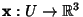be a Regular Patch, then the Shape Operatorof x is given in terms of the basis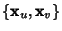by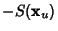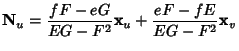(1)(2)

where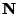is the Normal Vector,,, andthe coefficients of the first Fundamental Form(3)

and,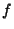, andthe coefficients of the second Fundamental Form given by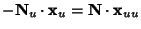(4)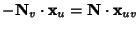(5)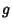(6)

Gray, A. Calculation of the Shape Operator.'' §14.3 in Modern Differential Geometry of Curves and Surfaces. Boca Raton, FL: CRC Press, pp. 274-277, 1993.